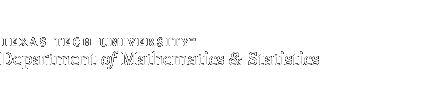Site Index

# Minor in Mathematics

## All of the following core courses are required:

• MATH 1451 - Calculus I
• MATH 1452 - Calculus II
• MATH 2450 - Calculus III

## One of the following two courses is required:TEST

• MATH 2360 - Linear Algebra
• MATH 3351 - Higher Mathematics for Engineers and Scientists II

## Six semester hours of mathematics at the junior-senior levelwhich can be selected from the following list are also required:

• MATH 3310 - Introduction to Mathematical Reasoning and Proof
• MATH 3342 - Mathematical Statistics for Engineers & Scientists  +
• MATH 3350 - Higher Mathematics for Engineers and Scientists I
or MATH 3354 - Differential Equations I
(credit may not be received for both 3350 and 3354)
• MATH 3360 - Foundations of Algebra I
• MATH 3430 - Computational Techniques for Science and Mathematics
• MATH 4000 - Selected topics in upper division Mathematics
• MATH 4310 - Introduction to Numerical Analysis I
• MATH 4312 - Introduction to Numerical Analysis II
• MATH 4330 - Mathematical Computing
• MATH 4342 - Mathematical Statistics I  +
• MATH 4343 - Mathematical Statistics II
• MATH 4350 - Advanced Calculus I
• MATH 4351 - Advanced Calculus II
• MATH 4354 - Differential Equations II
• MATH 4356 - Elementary Functions of Complex Variables
• MATH 4360 - Foundations of Algebra II
• MATH 4362 - Theory of Numbers
• MATH 4363 - Introduction to Combinatorics
+  MATH 3342 & 4342 cannot both be counted toward a Math Minor